International
Tables for
Crystallography
Volume B
Reciprocal space
Edited by U. Shmueli

International Tables for Crystallography (2006). Vol. B. ch. 4.5, pp. 470-471   | 1 | 2 |

## Section 4.5.2.4.3. Random copolymers

R. P. Millanea*

#### 4.5.2.4.3. Random copolymers

| top | pdf |

Random copolymers are made up of a small number of different kinds of monomer, whose sequence along the polymer chain is not regular, but is random, or partially random. A particularly interesting class are synthetic polymers such as copolyesters that form a variety of liquid-crystalline phases and have useful mechanical properties (Biswas & Blackwell, 1988a). The structures of these materials have been studied quite extensively using X-ray fibre diffraction analysis. Because the molecules do not have an average c repeat, their diffraction patterns do not consist of equally spaced layer lines. However, as a result of the small number of distinct spacings associated with the monomers, diffracted intensity is concentrated about layer lines, but these are irregularly spaced (along Z) and are aperiodic. Since the molecule is not periodic, the basic theory of diffraction by helical molecules described in Section 4.5.2.3.2does not apply in this case. Cylindrically averaged diffraction from random copolymers is described here. Related approaches have been described independently by Hendricks & Teller (1942)and Blackwell et al. (1984). Hendricks & Teller (1942)considered the rather general problem of diffraction by layered structures made up of different kinds of layers, the probability of a layer at a particular level depending on the layers present at adjacent levels. This is a one-dimensional disordered structure that can be used to describe a random copolymer. Blackwell and co-workers have developed a similar theory in terms of a one-dimensional paracrystalline model (Hosemann & Bagchi, 1962) for diffraction by random copolymers (Blackwell et al., 1984; Biswas & Blackwell, 1988a), and this is the theory described here.

Consider a random copolymer made up of monomer units (residues) of N different types. Since the disorder is along the length of the polymer, some of the main characteristics of diffraction from such a molecule can be elucidated by studying the diffraction along the meridian of the diffraction pattern. The meridional diffraction is the intensity of the Fourier transform of the polymer chain projected onto the z axis and averaged over all possible monomer sequences. The diffraction pattern depends on the monomer (molar) compositions, denoted by, the statistics of the monomer sequence (described by the probability of the different possible monomer pairs in this model) and the Fourier transform of the monomer units. Development of this model shows that the meridional diffracted intensity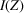can be written in the form (Blackwell et al., 1984; Biswas & Blackwell, 1988a; Schneider et al., 1991)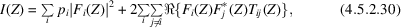where the summations are over the different monomer types and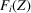is the axial Fourier transform of the ith monomer unit (each referenced to a common origin). The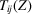are most conveniently described by defining them as the ijth element of anmatrix T, which is given by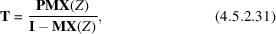where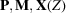and I arematrices. I is the unit matrix and P is a diagonal matrix with elements. The elements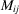of M are the probabilities of forming ij monomer pairs and can be generated for different kinds of random sequence (e.g. chemical restrictions on the occurrence of particular monomer pairs, random chains, varying degrees of blockiness, tendency towards alternating sequences etc.) (Schneider et al., 1991). The matrix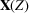is diagonal with elements equal to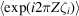where the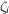are the projected monomer lengths and the average is over all chain conformations.

Equation (4.5.2.30)can be used to calculate the meridional diffraction for a particular random copolymer. The most important result of such a calculation is that intensity maxima are spaced irregularly along the meridian. The positions of the maxima depend on the monomer proportions, the sequence statistics and the projected monomer lengths.

The full cylindrically averaged diffraction pattern, denoted by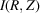, from a noncrystalline specimen containing oriented random copolymer chains can be calculated by replacingin equation (4.5.2.30)by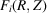, i.e.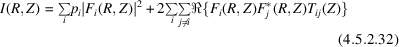(Biswas & Blackwell, 1988a). Note that we writerather than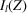since the pattern cannot be indexed on the basis of regularly spaced layer lines. Thein equation (4.5.2.32)depend on the chain conformation, since this affects the range of monomer orientations and hence their average diffraction. Chivers & Blackwell (1985)have considered two extreme cases, one corresponding to fixed conformations between monomers and the other corresponding to completely random conformations between monomers, and have derived expressions for the diffracted intensity in both cases. Equation (4.5.2.32)allows one to calculate the fibre diffraction pattern from an array of parallel random copolymers that exhibit no lateral ordering. The diffraction pattern consists of irregularly spaced layer lines whose spacings (in Z) are the same as those described above for the meridional maxima. Measurement of layer-line spacings and intensities and comparison with calculations based on the constituent monomers allows chain conformations to be estimated (Biswas & Blackwell, 1988a).

Diffraction patterns from liquid-crystalline random copolymers often contain sharp Bragg maxima on the layer lines. This indicates that, despite the random sequence and the possible dissimilarity of the component monomers, the chains are able to pack together in a regular way (Biswas & Blackwell, 1988b,c). Expressions that allow calculation of diffraction patterns for arrays of polymers with minimal registration, in which short, non-identical sequences form layers, have been derived (Biswas & Blackwell, 1988b,c). Calculation of diffracted intensities, coupled with molecular-mechanics modelling, allows chain conformations and packing to be investigated (Hofmann et al., 1994).

### ReferencesBiswas, A. & Blackwell, J. (1988a). Three-dimensional structure of main-chain liquid-crystalline copolymers. 1. Cylindrically averaged intensity transforms of single chains. Macromolecules, 21, 3146–3151.Google ScholarBiswas, A. & Blackwell, J. (1988b). Three-dimensional structure of main-chain liquid-crystalline copolymers. 2. Interchain interference effects. Macromolecules, 21, 3152–3158.Google ScholarBiswas, A. & Blackwell, J. (1988c). Three-dimensional structure of main-chain liquid-crystalline copolymers. 3. Chain packing in the solid state. Macromolecules, 21, 3158–3164.Google ScholarBlackwell, J., Gutierrez, G. A. & Chivers, R. A. (1984). Diffraction by aperiodic polymer chains: the structure of liquid crystalline copolyesters. Macromolecules, 17, 1219–1224.Google ScholarChivers, R. A. & Blackwell, J. (1985). Three-dimensional structure of copolymers of p-hydroxybenzoic acid and 2-hydroxy-6-naphthoic acid: a model for diffraction from a nematic structure. Polymer, 26, 997–1002.Google ScholarHendricks, S. & Teller, E. (1942). X-ray interference in partially ordered layer lattices. J. Chem. Phys. 10, 147–167.Google ScholarHofmann, D., Schneider, A. I. & Blackwell, J. (1994). Molecular modelling of the structure of a wholly aromatic thermotropic copolyester. Polymer, 35, 5603–5610.Google ScholarHosemann, R. & Bagchi, S. N. (1962). Direct analysis of diffraction by matter. Amsterdam: North-Holland.Google ScholarSchneider, A. I., Blackwell, J., Pielartzik, H. & Karbach, A. (1991). Structure analysis of copoly(ester carbonate). Macromolecules, 24, 5676–5682.Google Scholar